In this page we have NCERT book Solutions for Class 9th Maths Chapter 8 Quadrilaterals for EXERCISE 8.2 . Hope you like them and do not forget to like , social share and comment at the end of the page.

## Chapter 8 Ex 8.2

Question 1.
ABCD is a quadrilateral in which P, Q, R and S are mid-points of the sides AB, BC, CD and DA. AC is a diagonal. Show that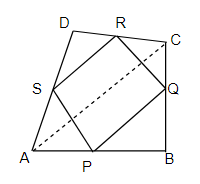(i) SR || AC and SR =1/2 AC
(ii) PQ = SR
(iii) PQRS is a parallelogram
Solution
(i) In Δ ACD
S is the mid point of AD
R is the mid point of CD
By Mid point theorem
SR || AC and SR =1/2 AC --(X)

(ii)
In Δ ABC
P is the mid point of AB
Q is the mid point of BC
By Mid point theorem
PQ || AC and PQ =1/2 AC --(Y)
From equation X and Y
PQ = SR
and PQ || SR||AC

PQ=SR
and PQ||SR
Hence PQRS is a parallelogram

Question 2.
ABCD is a rhombus and P, Q, R and S are the mid points of sides AB, BC, CD and DA respectively. Show that the quadrilateral PQRS is a rectangle.
Solution
The figure is shown as below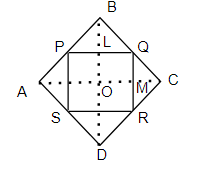To Prove: quadrilateral PQRS is a rectangle
Proof:
In Δ ABC
P and Q are mid points of sides AB and BC
By Mid point theorem
PQ= 1/2 AC and PQ || AC -(X)

In Δ ACD
S and R are mid points of sides AD and DC
By Mid point theorem
SR= 1/2 AC and SR || AC --(Y)
From (X) and (Y), we have
PQ=SR
PQ ||SR
Hence PQSR is a parallelogram

Now in Δ BCD
Q and R are mid points of sides BC and DC
By Mid point theorem
BD || QR
or
QM || OL

PQ ||SR
or LQ ||OM

In LQOM
QM || OL
LQ ||OM
Therefore LQOM is a parallelogram
Now,we know that opposite angles in the parallelogram are equal
∠ LQM = ∠ LOM
Now ∠ LOM=90 as diagonals bisects at right angle in Rhombus
Therefore
∠ LQM=90°
or
∠ PQR=90°
Now
PQRS is a parallelogram with one angle as right angle. Hence PQRS is a rectangle

Question 3.
ABCD is a rectangle and P, Q, R and S are mid points of the sides AB, BC, CD and DA respectively. Show that quadrilateral PQRS is a rhombus.
Solution
The figure is shown as below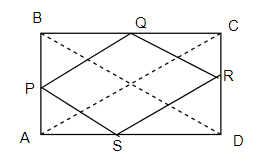To Prove: quadrilateral PQRS is a rhombus
Proof:
In Δ ABC
P and Q are mid points of sides AB and BC
By Mid point theorem
PQ= 1/2 AC and PQ || AC -(X)

In Δ ACD
S and R are mid points of sides AD and DC
By Mid point theorem
SR= 1/2 AC and SR || AC --(Y)
From (X) and (Y), we have
PQ=SR
PQ ||SR
Hence PQSR is a parallelogram

Now in Δ BCD
Q and R are mid points of sides BC and DC
By Mid point theorem
QR= 1/2 BD
Nowe AC = BC
Hence
PQ=SR=QR
Now a parallelogram whose adjacent sides are equal is a rhombus.
Hence proved

Question 4.
ABCD is a trapezium in which AB || DC, BD is a diagonal and E is the mid-point of AD. A line is drawn through E parallel to AB intersecting BC at F . Show that F is the mid-point of BC.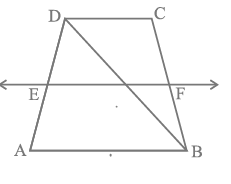Solution
Let G be the point on BD where EF intersect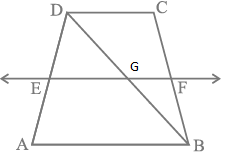Now in Δ ABD
E is the mid-point of AD
EG ||AB
By converse of Mid-point theorem
G is the mid-point of BD

Now in Δ BDC
G is the mid-point of BD
FG || BC
By converse of Mid-point theorem
G is the mid-point of BC

Question 5.
In a parallelogram ABCD, E and F are the mid-points of sides AB and CD respectively. Show that the line segments AF and EC trisect the diagonal BD.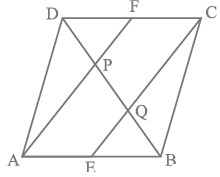Solution
AB=CD ( Opposite side of parallelogram)
Since E and F are mid-points of AB and DC respectively.
AE = 1/2 AB and CF = 1/2 DC
Therefore
AE = CF and AE || CF.
One pair of opposite sides is parallel and equal
Hence AECF is a parallelogram.
AF ||EC
In Δ BAP
E is the mid-point of AB
Now AF ||EC
So, EQ || AP
Now Converse of mid-point theorem
Q is mid-point of PB
PQ = QB ---(X)
Similarly, in ΔDQC, P is the mid-point of DQ
DP = PQ ---(Y)
From (X) and (Y), we have DP = PQ = QB

Question 6.
Show that the line segments joining the mid- point of the opposite sides of a quadrilateral bisect each other.
Solution
To Prove : EG and FH bisect each other.
Construction : Join EF, FG, GH, HE and AC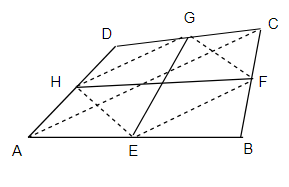Proof:
In Δ ABC
E and F are mid-points of AB and BC respectively.
By Mid point theorem
EF = 1/2 AC and EF || AC ----(i)
H and G are mid-points of AD and CD respectively.
By Mid point theorem
HG = 1/2 AC and HG || AC ----(ii)
From (i) and (ii), we get
EF = HG
and EF || HG
Hence EFGH is a parallelogram
Now We know that Diagonals of the parallelogram bisects each other
Here EG and FH are diagonals of the parallelogram EFGH.
Hence Proved

Question 7.
ABC is a triangle right angles at C. A line through the mid -point M of hypotenuse AB and parallel to BC intersects AC at D. Show that
1. D is mid- point of AC
2. MD ⊥ AC
3. $CM = MA = \frac {1}{2} AB$
Solution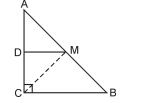Construction : Join CM.
Proof:
(i) In Δ ABC
M is the mid-point of AB.
BC || DM
By converse of Mid-point theorem
D is the mid-point of AC

(ii)
Now BC || DM
Hence Corresponding angles]
Now ∠ACB=90°
Hence
Hence MD ⊥ AC

(iii) In Δ ADM and CMD
∠ ADM = ∠ CDM = 90°
DM is common
Therefore by SAS congruence
Now by CPCT
CM=MA
Since M is mid-point of AB,
MA = 1/2 AB
Hence, $CM = MA = \frac {1}{2} AB$

## Summary

1. NCERT solutions for class 9 maths chapter 8 Quadrilaterals Exercise 8.2 has been prepared by Expert with utmost care. If you find any mistake.Please do provide feedback on mail. You can download the solutions as PDF in the below Link also
2. This chapter 8 has total 2 Exercise 8.1 and 8.2. This is the Last exercise in the chapter.You can explore previous exercise of this chapter by clicking the link below